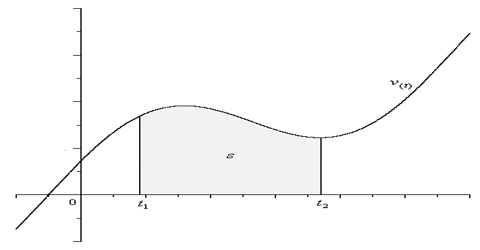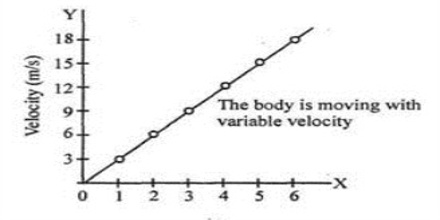Physics

# Variable Velocity Related to MotionVariable velocity: If the velocity of a body is different at different times then that velocity is called variable velocity. So if the magnitude or direction or both of rates of change of displacement with time changes then that rate of change of displacement is called variable velocity.

Explanation:

A body is said to be moving with variable velocity if it covers distances in uneven intervals of time or irregular distances in equal intervals of time, however small these intervals may be, or it changes its direction of motion.Let a body travel 5 m in the first second, 8 m in the second second and 7 m in the third second in a particular direction [Fig (a)]. Here the body is not travelling the same distance in the same interval of time. So this velocity is variable velocity. The graph of variable velocity is shown in fig (b).

A body has uniform velocity of 5 ms-1. It means that in a particular direction the body travels 5 m in every second.